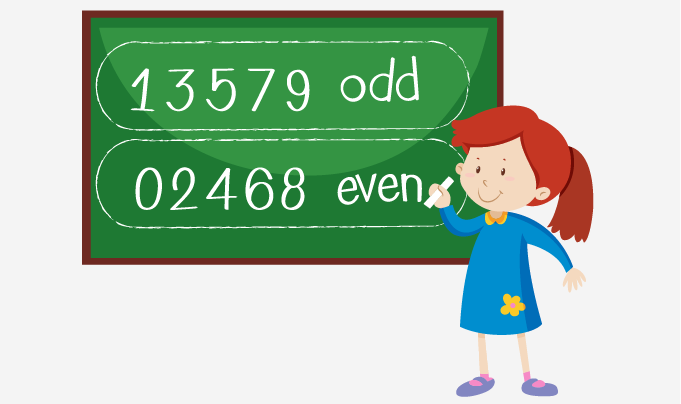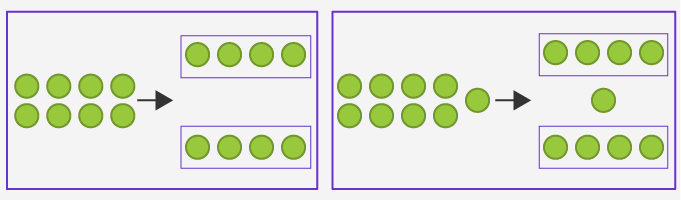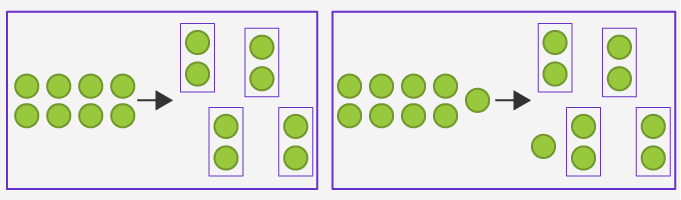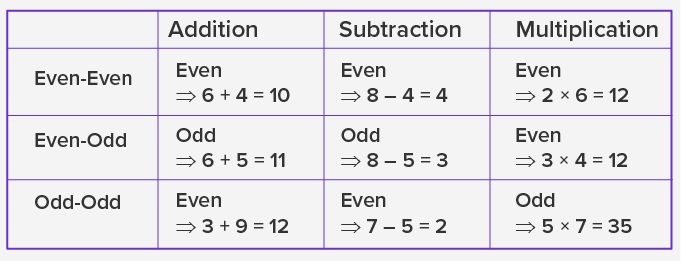# Even And Odd Numbers - Definition with Examples

The Complete K-5 Math Learning Program Built for Your Child

• 40 Million Kids

Loved by kids and parent worldwide

• 50,000 Schools

Trusted by teachers across schools

• Comprehensive Curriculum

Aligned to Common Core

## Even and Odd Numbers

A number which is divisible by 2 and generates a remainder of 0 is called an even number. An odd number is a number which is not divisible by 2. The remainder in the case of an odd number is always “1”.

The property by which we classify an integer in math as even or odd is also known as parity.

## Identifying even or odd number

1. By comprehending the number at “ones” place

In this approach, we analyze the number at “ones” place in an integer to check if the number is even or odd.

All the numbers ending with 1,3,5,7 and 9 are odd numbers. For example, numbers such as 11, 23, 35, 47 etc. are odd numbers.

All the numbers ending with 0,2,4,6 and 8 are even numbers. For example, numbers such as 14, 26, 32, 40 and 88 are even numbers.

 25 , 32, 38, 87, 95, 64, 76, 53 Even Odd 32, 38, 64, 76 25, 87, 95, 532. By grouping

• As two equal groups

If we divide a number into two groups with an equal number of elements in each, then the number is an even number. In the case of odd numbers, we get a remainder of 1 while grouping.• As groups of “two” in each

For a number, if it forms multiple groups of “two” without any remainder, it is an even number. In the case of a remainder, a number is an odd number.The given table explains the result when we apply different operations on a set of two numbers.Application

The elementary skills in number sense are useful in later grades for learning math, science, and communication systems. We apply the concept in designing circuits using logic gates and binary codes. In ancient mathematics, the learning of geometric shapes started from categorizing the shapes based on the number of sides as even and odd.

 Fun Facts Each alternate number in the counting is an even number starting from 2 and an odd number starting from 1. Zero is an even number Ancient Greeks used shapes and figures with an odd number of sides to represent “odd” numbers Pythagoreans used the term “gnomon” for the odd numbers

Won Numerous Awards & Honors